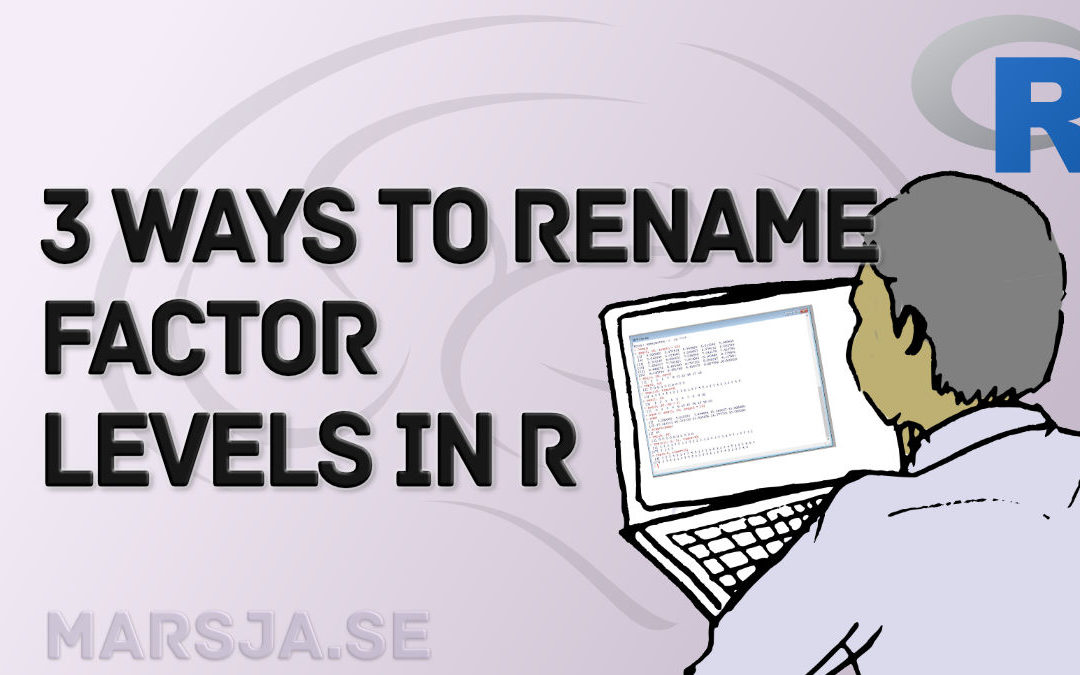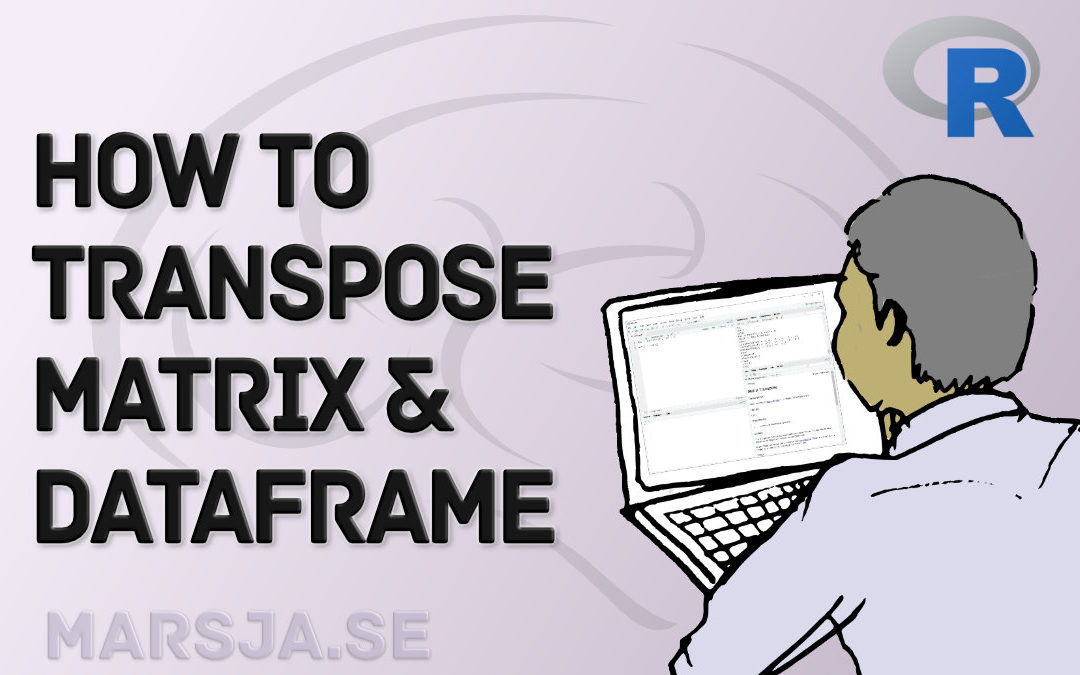## How to Rename Factor Levels in R using levels() and dplyr

In this tutorial, you will learn how to rename factor levels in R. First, we will use the base functions that are available in R, and then we will use dplyr.  To rename factor levels using levels() we can assign a character vector with the new names. If we want...## How to Remove Duplicates in R – Rows and Columns (dplyr)

In this R tutorial, you will learn how to remove duplicates from the data frame. First, you will learn how to delete duplicated rows and, second, you will remove columns. Specifically, we will have a look at how to remove duplicate records from the data frame using 1)...## Levene’s & Bartlett’s Test of Equality (Homogeneity) of Variance in Python

In this Python tutorial, you will learn how to 1) perform Bartlett’s Test, and 2) Levene’s Test. Both are tests that are testing the assumption of equal variances. Equality of variances (also known as homogeneity of variance, and homoscedasticity) in population...## R: Add a Column to Dataframe Based on Other Columns with dplyr

In this R tutorial, you are going to learn how to add a column to a dataframe based on values in other columns. Specifically, you will learn to create a new column using the mutate() function from the package dplyr, along with some other useful functions. Finally, we...## How to use %in% in R: 7 Example Uses of the Operator

In this tutorial, you will learn by examples how to use the %in% in R. Specifically, you will learn 7 different uses of this great operator. Outline Here’s the outline of this post, described a bit more detailed than the table of contents. First, we start out with a...## How to Transpose a Dataframe or Matrix in R with the t() Function

In this brief tutorial, you will learn how to transpose a dataframe or a matrix in R statistical programming environment. Transposing rows and columns is a quite simple task if your data is 2-dimensional (e.g., a matrix or a dataframe). If you have a, for example,...